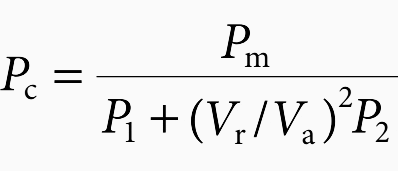﻿ Transformer no-load loss and excitation-current measurements | EEP

# Transformer no-load loss and excitation-current measurements

Home / Technical Articles / Transformer no-load loss and excitation-current measurements

## Purpose of no-load loss measurements

A transformer dissipates a constant no-load loss as long as it is energized at constant voltage, 24 h a day, for all conditions of loading. This power loss represents a cost to the user during the lifetime of the transformer.

Maximum values of the no-load loss of transformers are specified and often guaranteed by the manufacturer. No-load-loss measurements are made to verify that the no-load loss does not exceed the specified or guaranteed value.

### Average-voltage voltmeter method

Transformer no-load loss, often called core loss or iron loss, is the power loss in a transformer excited at rated voltage and frequency but not supplying load.

The no-load loss comprises three components:

1. Core loss in the core material
2. Dielectric loss in the insulation system
3. I2R loss due to excitation current in the energized winding

The no-load loss of a transformer is primarily caused by losses in the core steel. The remaining two sources are sometimes ignored. As a result, the terms no-load loss, core loss, and iron loss are often used interchangeably. Core loss and iron loss, strictly speaking, refer only to the power loss that appears within the core material.

The following discussion on no-load loss, or core loss, will explain why the average-voltage voltmeter method, to be described later, is recommended.

The magnitude of no-load loss is a function of the magnitude, frequency, and waveform of the impressed voltage. These variables affect the magnitude and shape of the core magnetic flux waveform and hence affect the value of the core loss.

It has been verified through measurements on power and distribution transformers that core loss also depends, to some extent, upon the temperature of the core.

According to the IEEE, ‘Standard Test Code for Liquid-Immersed Distribution, Power, and Regulating Transformers and Guide for Short-Circuit Testing of Distribution and Power Transformers’, the approximate rate of change of no-load loss with core temperature is 0.00065p.u. core loss increase for each °C reduction in core temperature.

The two main components of the core loss are hysteresis loss and eddy-current loss.

The change in eddy-current loss, due to a change in the resistivity of the core steel as temperature changes, appears to be one factor that contributes to the observed core-loss temperature effect. The hysteresis loss magnitude is a function of the peak flux density in the core-flux waveform.

When the impressed voltage waveform is distorted (not a pure sine wave), the resulting peak flux density in the flux waveform depends on the average absolute value of the impressed voltage wave.

Eddy-current loss is a function of the frequency of the power source and the thickness of the core-steel laminations. Eddy loss is strongly influenced by harmonics in the impressed voltage.

The above mentioned IEEE transformer test code recommends the average-voltage voltmeter method, to be described below, for measuring no-load loss.

Excitation current is the current flowing into the high voltage winding with the low voltage side open. This current should be proportional to the No-load acceptance test but with the difference resultant from the use of test voltages different from nominal values.

It shouldn’t exist excessive deflection from values measured between phases and its normal a slight difference (geometric) between extreme and central windings. It will exist great changes when appear heat points, degradation in the magnetic package, loose core or detached magnetic shunt.

### How no-load loss is measured

#### Average-voltage voltmeter method

The measurement of no-load loss, according to the average-voltage voltmeter method, is illustrated in Figure 1. Voltage and current transformers are required to scale the inputs for voltmeters, ammeters, and wattmeters.

Three-phase no-load-loss measurements are carried out the same way, except that three sets of instruments and instrument transformers are utilized.

The test involves raising voltage on one winding, usually the low-voltage winding, to its rated voltage while the other windings are in open circuit. Two voltmeters connected in parallel are employed.

The voltmeter labeled Va in Figure 1 represents an average-responding, rms-calibrated voltmeter. The voltmeter labeled Vr represents a true rms-responding voltmeter.

Harmonics in the impressed voltage will cause the rms value of the waveform to be different from the average-absolute (rms-scaled) value, and the two voltmeter readings will differ. When the voltage reading, as measured by the average-responding voltmeter, reaches a value corresponding to the rated voltage of the excited winding, readings are taken of the rms current, the rms voltage, and the no-load power.

The ratio of the measured rms current to the rated load current of the excited winding, expressed in percent, is commonly referred to as the percent excitation current. The measured no-load loss is corrected to a sine-wave basis by a formula given in the mentioned IEEE test code, using the readings of the two voltmeters.

The correction is shown below. The corrected value is reported as the no-load loss of the transformer.where:

• Pc is the corrected (reported) value of no-load loss
• Pm is the measured value of no-load loss
• Va is the reading of the average-responding, rms-calibrated voltmeter
• Vr is the reading of the true-rms-responding voltmeter
• P1 and P2 are the per-unit hysteresis and per-unit eddy-current losses, respectively

According to the mentioned IEEE test code, if the actual values of P1 and P2 are not available, it is suggested that the two loss components be assumed equal in value, assigning each a value of 0.5 p.u..

Sources:

1. The Electric Power Engineering Handbook by Leonard L. Grigsby (Purchase hardcover from Amazon)
2. Power transformer maintenance – Field Testing by Andrés Tabernero García### Edvard Csanyi

Electrical engineer, programmer and founder of EEP. Highly specialized for design of LV/MV switchgears and LV high power busbar trunking (<6300A) in power substations, commercial buildings and industry fascilities. Professional in AutoCAD programming.

1.2.